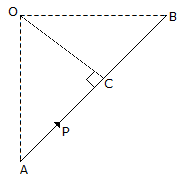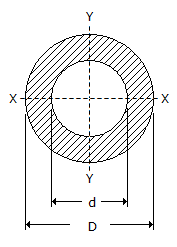Last updated: August 14, 2020

1. When the lift is moving upwards with some acceleration, the pressure exerted by a man is __________ proportional to its acceleration.
2. The moment of the force P about O as shown in the below figure is3. If a body is acted upon by a number of coplaner non-concurrent forces, it may
4. The maximum mechanical advantage of a lifting machine is
5. The centre of gravity a T-section 100 mm x 150 mm x 50 mm from its bottom is
6. When a body falls freely under gravitational force, it possesses __________ weight.
7. An ideal machine is one whose efficiency is
8. The centre of gravity of a quadrant of a circle lies along its central radius (r) at a distance of
9. According to Lami's theorem
10. A machine having an efficiency greater than 50%, is known as
11. Moment of inertia is the
12. Moment of inertia of a hollow circular section, as shown in the below figure about an axis perpendicular to the section, is __________ than that about X-X axis.13. The horizontal range of a projectile (R) is given by
14. In order to completely specify angular displacement by a vector, it must fix
15. The coefficient of restitution for inelastic bodies is
16. The impact between two lead spheres is approximately equal to an __________ impact.
17. Which of the following are vector quantities?
18. The energy possessed by a body, for doing work by virtue of its position, is called
19. Which of the following statement is correct in connection with projectiles?
20. Moment of inertia of a triangular section of base (b) and height (h) about an axis passing through its vertex and parallel to the base, is __________ than that passing through its C.G. and parallel to the base.
Engineering Mechanics MCQ 12
You got {{userScore}} out of {{maxScore}} correct
{{title}}
{{image}}
{{content}}